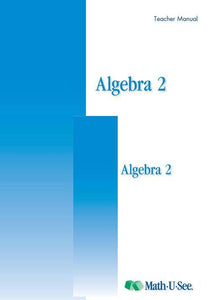or 6 weekly interest-free payments from \$14.83 withwhat's this?Demme Learning

# Math.U.See Algebra 2

Regular price \$20.00 \$51.38 Unit price per
Tax included. Shipping calculated at checkout.

## Math.U.See Algebra 2

#### Factoring polynomials, quadratic formula, graphing conic sections and other algebra 2 topics.

Major Concepts and Skills Include:

• Simplifying multiple-degree rational expressions
• Working with imaginary and complex numbers
• Understanding and applying the binomial theorem
• Solving basic quadratic equations with factoring
• Applying analytic geometry to conic sections
• Solving simultaneous equations (linear and conic) using graphing, substitution, and elimination

• Applying basic algebra to problems involving ratios and proportions
• Graphing inequalities
• Using the distance and midpoint formulas
• Solving equations with three variables
• Solving problems involving distance, rate, and time
• Understanding vectors

Algebra 2 Instruction Pack includes: Instruction Manual & 2xDVDs

Algebra 2 Student pack includes: Student Workbook & Test & Activity booklet.

Honour Textbook: provides extension work & extra practice

Grab an extra Student Pack for an additional Student.